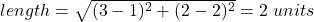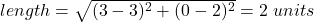## Find the area of the polygon with the coordinates (1, 2), (3, 2), (3, 0), and (1,0). 2 sq. units 8 sq. units 4 sq. u

Question

Find the area of the polygon with the coordinates (1, 2), (3, 2), (3, 0), and
(1,0).
2 sq. units
8 sq. units
4 sq. units
16 sq. units

in progress 0
7 months 2021-07-28T05:26:34+00:00 1 Answers 2 views 0

4 sq. units

Step-by-step explanation:

Since the polygon has 4 vertices, hence the polygon is a quadrilateral with four sides and four angles.

We can see that for this polygon, opposite sides are parallel and equal to each other.

To find the area of the polygon, we have to first get the length of the polygon and then the width of the polygon, hence:

The length is the distance between (1, 2) and (3, 2):The breadth is the distance between (3, 2) and (3, 0):Since length = breadth, hence this is a square.

Area= length * breadth = 2 * 2 = 4 sq. units